Open in App
Not now

# Servlet – forward() and sendRedirect() Method With Example

• Last Updated : 27 Dec, 2021

As we learned in the previous topics, Servlets are the Java programs that run on the server-side and generate dynamic responses to the client request. The execution of the Servlet involves the following steps:

• The client sends the request through the browser to the Web server/Application Server.
• The server passes the client request to the respective servlet.
• Servlet processes the request generates the response and sends the response back to the server.
• The server passes the generated response to the browser/client.

Now, let’s consider we have a requirement to call a servlet from another servlet bypassing the information using request and response objects. We can achieve this in 2 ways, servlet provides

• forward() method in RequestDispatcher(I).
• sendRedirect() method in HttpServletResponse(I)

### RequestDispatcher Interface

Servlets provide RequestDispatcher in javax.servlet package. It defines an object to dispatch the client request from one servlet to another servlet on the same server. Basically, this can be used to receive the client request object and send that object to any other resource such as Servlet, JSP, HTML file, etc. We can get the RequestDispatcher object using the below syntax:

## Java

 `RequestDispatcher r = req.getRequestDispatcher(String arg);`

### forward() method

This method forwards a request from a servlet to another servlet on the same server. It allows one servlet to do the initial processing of a request, obtains the RequestDispatcher object, and forwards the request to another servlet to generate the response.

Syntax of forward():

## Java

 `// r - requestDispatcher object ` `r.forward(req, resp);`

When we are forwarding the client request using the forward() method, the client/browser doesn’t even know that the request is forwarding to another servlet file or JSP file or from which file response will be generating. There will be no change in the URL in the browser.

### sendRedirect() method

It sends a temporary redirect response to the client using the specified redirect location URL given in the method and clears the buffer. Let’s consider a scenario, where we need to redirect the request from a servlet to other servlets which is in another server, in this case, we can use the sendRedirect() method so that the client/browser knows where the request is going and getting processed.

Syntax to use sendRedirect():

## Java

 `resp.sendRedirect(java.lang.String location)`

Let’s take an example to understand more about these two methods.

Example:

Consider a simple example – Take two integer values from the client and generate the sum and average of those two numbers. To demonstrate the use of forward() and sendRedirect() methods, we will be performing addition operations in one servlet and dispatches that request object to another servlet to perform the average operation.

### Program 1: Using forward() method

Steps:

• Create a simple HTML page to take the values from the browser.
• Create the first servlet to get the request and perform an addition operation.
• Create a second servlet to perform the average operation and send the response.

Step 1: Create a simple HTML page to take the values from the browser

Home.html

## HTML

 ` ` `<``html``> ` `<``head``> ` `<``meta` `charset``=``"ISO-8859-1"``> ` `<``title``>Home Page ` ` ` `<``body``> ` ` `  `<``h3``>Enter two numbers to find their Sum and Average ` ` `  `    ``<``form` `action``=``"add"` `method``=``"get"``> ` `        ``` `        ``First Number: <``input` `type``=``"text"` `name``=``"x"``> ` `        ``<``br``/> ` `        ``` `        ``Second Number: <``input` `type``=``"text"` `name``=``"y"``> ` `        ``<``br``/> ` ` `  `        ``<``input` `type``=``"submit"``> ` `    `` ` ` `  ` ` ``

Use input tags to take the values from the client, say x, y. We have a form tag that will map the servlet based on the action attribute – “add” and to the “doGet” method in the servlet when submits the page.

Step 2: Create the first servlet to get the request and perform an additional operation

## Java

 `import` `java.io.IOException; ` ` `  `import` `javax.servlet.RequestDispatcher; ` `import` `javax.servlet.ServletException; ` `import` `javax.servlet.annotation.WebServlet; ` `import` `javax.servlet.http.HttpServlet; ` `import` `javax.servlet.http.HttpServletRequest; ` `import` `javax.servlet.http.HttpServletResponse; ` ` `  `@WebServlet``(``"/add"``) ` `public` `class` `AddNum ``extends` `HttpServlet { ` ` `  `    ``@Override` `    ``protected` `void` `doGet(HttpServletRequest req, HttpServletResponse resp) ``throws` `ServletException, IOException { ` ` `  `        ``// Get the x, y parameters from the request  ` `        ``// object and save in num1, num2 variables. ` `        ``int` `num1 = Integer.parseInt(req.getParameter(``"x"``)); ` `        ``int` `num2 = Integer.parseInt(req.getParameter(``"y"``)); ` ` `  `        ``// Perform addition operation on num1, num2 ` `        ``// and save the result in add variable. ` `        ``int` `add = num1 + num2; ` `         `  `        ``// Set the add value in 'sum'  ` `        ``// attribute of request object ` `        ``req.setAttribute(``"sum"``, add); ` ` `  `        ``// Get the Request Dispatcher object and pass  ` `        ``// the argument to which servlet we need to call - AvgNum.java ` `        ``RequestDispatcher reqd = req.getRequestDispatcher(``"avg"``); ` `       `  `        ``// Forward the Request Dispatcher object. ` `        ``reqd.forward(req, resp); ` `    ``} ` ` `  `}`

• In this servlet, we are taking the input values from the request object and performing the addition operation.
• Now, to call another servlet to perform the average operation, we need to pass the ‘add’ value to that servlet.
• So, by using setAttribute, we need to set the ‘sum’ attribute with the ‘add’ value.
• Finally, get the Request Dispatcher object and pass the argument and forward the object. In this example, we mentioned AddNum.java as ‘/add’ and AvgNum.java as ‘/avg’.

Step 3: Create a second servlet to perform the average operation and send the response.

AvgNum.java

## Java

 `import` `java.io.IOException; ` `import` `java.io.PrintWriter; ` ` `  `import` `javax.servlet.ServletException; ` `import` `javax.servlet.annotation.WebServlet; ` `import` `javax.servlet.http.HttpServlet; ` `import` `javax.servlet.http.HttpServletRequest; ` `import` `javax.servlet.http.HttpServletResponse; ` ` `  `@WebServlet``(``"/avg"``) ` `public` `class` `AvgNum ``extends` `HttpServlet { ` ` `  `    ``@Override` `    ``protected` `void` `doGet(HttpServletRequest req, HttpServletResponse resp) ``throws` `ServletException, IOException { ` ` `  `        ``// Get the 'sum' attribute value from the request ` `        ``int` `sum = (``int``) req.getAttribute(``"sum"``); ` ` `  `        ``// perform the average operation and  ` `        ``// save the result in 'avg' variable. ` `        ``float` `avg = (``float``) (sum / ``2.0``); ` `         `  `        ``// Get the PrintWriter object to write  ` `        ``// the output in the response to the browser. ` `        ``PrintWriter out = resp.getWriter(); ` `        ``out.println(``"Sum is: "` `+ sum); ` `        ``out.println(``"Average is: "` `+ avg); ` ` `  `    ``} ` ` `  `}`

• In this second servlet, get the request object ‘sum’, which we set in the first servlet.
• Perform the average operation and by using the PrintWriter object, output the result to the client in the response.

Execution:

• Run the Home.html page using ‘Run As -> Run on Server’ in Eclipse.
• Run the URL: http://localhost:8081/ServletCall/Home.html, in the browser to get the home page.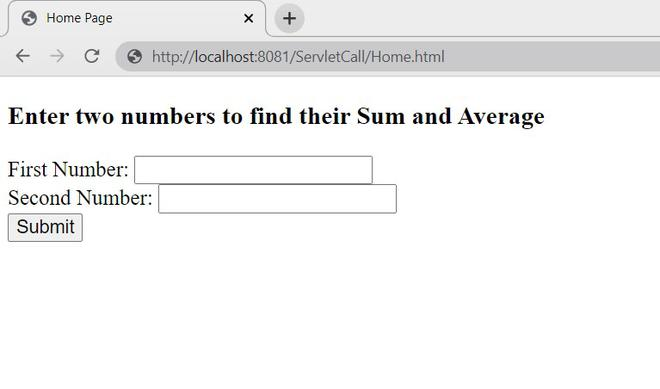Home.html

• Enter the values and click on submit.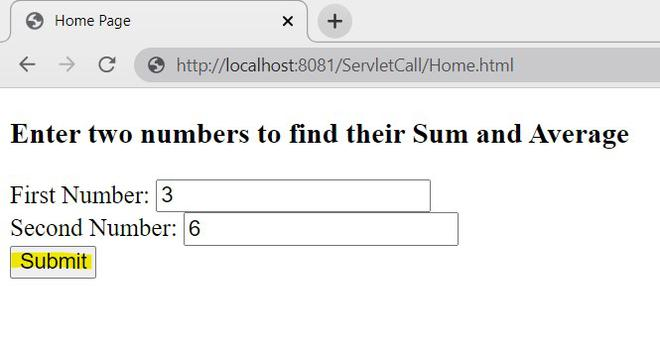• Once the page is submitted, the request will go to the ‘doGet’ method in the AddNum.java servlet, as we mentioned

## HTML

 `<``form` `action``=``"add"` `method``=``"get"``>`

• In the AddNum.java servlet, we specified the servlet with

## Java

 `@WebServlet``(``"/add"``)`

• So, the Home.html page maps to the AddNum.java servlet and sends the request.
• In AddNum.java, it performs the addition operation on the given 2 values and sets the added value in the request to send to the other servlet using RequestDispatcher.

## Java

 `// Get the Request Dispatcher object and pass the argument ` `// to which servlet we need to call - AvgNum.java ` `RequestDispatcher reqd = req.getRequestDispatcher(``"avg"``); ` `// Forward the Request Dispatcher object. ` `reqd.forward(req, resp);`

• Here, we are passing argument as ‘avg’, so it maps to the AvgNum.java servlet as we specified with

## Java

 `@WebServlet``(``"/avg"``)`

• In AvgNum.java, we will be getting the ‘sum’ value and performing the average operation.
• So, the output will be as follow,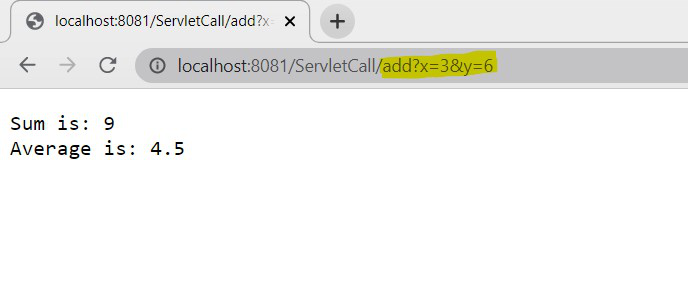Result – forward()

• If you observe the URL in the browser, it is showing the ‘/add’ servlet only. So the forward request done on the server-side does not affect the client-side.

### Program 2: Using sendRedirect() method

Now, we will use the sendRedirect() method instead of forward() in the same example.

• There is no need to change anything on the Home.html page.
• In the first servlet, instead of forward() method, use sendRedirect() method.
• In the second servlet, get the attribute from the request.

## Java

 `import` `java.io.IOException; ` ` `  `import` `javax.servlet.ServletException; ` `import` `javax.servlet.annotation.WebServlet; ` `import` `javax.servlet.http.HttpServlet; ` `import` `javax.servlet.http.HttpServletRequest; ` `import` `javax.servlet.http.HttpServletResponse; ` ` `  `@WebServlet``(``"/add"``) ` `public` `class` `AddNum ``extends` `HttpServlet { ` ` `  `    ``@Override` `    ``protected` `void` `doGet(HttpServletRequest req, HttpServletResponse resp) ``throws` `ServletException, IOException { ` ` `  `        ``// Get the x, y parameters from the request ` `        ``// object and save in num1, num2 variables. ` `        ``int` `num1 = Integer.parseInt(req.getParameter(``"x"``)); ` `        ``int` `num2 = Integer.parseInt(req.getParameter(``"y"``)); ` ` `  `        ``// Perform addition operation on num1,  ` `        ``// num2 and save the result in add variable. ` `        ``int` `add = num1 + num2; ` `         `  `        ``// Redirect the response to the ` `        ``// other servlet - AvgNum.java ` `        ``resp.sendRedirect(``"avg?sum="``+add); ` ` `  `    ``} ` ` `  `}`

• Here, we are using sendRedirect() to send the response to the other servlet bypassing the ‘add’ value.

AvgNum.java

## Java

 `import` `java.io.IOException; ` `import` `java.io.PrintWriter; ` ` `  `import` `javax.servlet.ServletException; ` `import` `javax.servlet.annotation.WebServlet; ` `import` `javax.servlet.http.HttpServlet; ` `import` `javax.servlet.http.HttpServletRequest; ` `import` `javax.servlet.http.HttpServletResponse; ` ` `  `@WebServlet``(``"/avg"``) ` `public` `class` `AvgNum ``extends` `HttpServlet { ` ` `  `    ``@Override` `    ``protected` `void` `doGet(HttpServletRequest req, HttpServletResponse resp) ``throws` `ServletException, IOException { ` ` `  `        ``// Get the 'sum' parameter from the request ` `        ``int` `sum = Integer.parseInt(req.getParameter(``"sum"``)); ` ` `  `        ``// perform the average operation and ` `        ``// save the result in 'avg' variable. ` `        ``float` `avg = (``float``) (sum / ``2.0``); ` `         `  `        ``// Get the PrintWriter object to write the ` `        ``// output in the response to the browser. ` `        ``PrintWriter out = resp.getWriter(); ` `        ``out.println(``"Sum is: "` `+ sum); ` `        ``out.println(``"Average is: "` `+ avg); ` ` `  `    ``} ` ` `  `}`

• Here we are getting the ‘sum’ value as a parameter from the request.

Execution:

• Same as above, run the program ‘Run on Server’.
• Run the URL, http://localhost:8081/ServletCall/Home.html in the browser.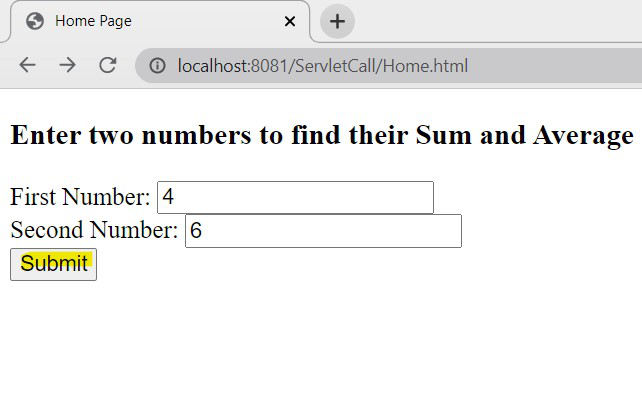• Enter the values and click on submit.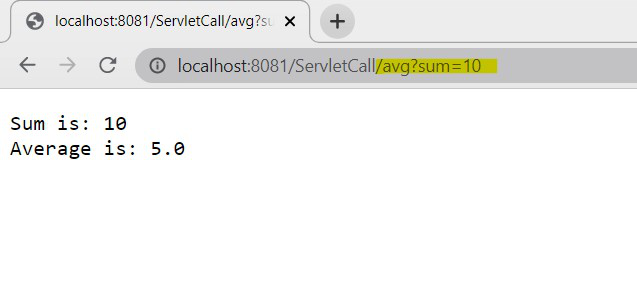• Now, if you observe the URL, we can see the request is redirected to another servlet with the sum value.
• The redirection of the request is visible on the browser side.

### Conclusion

When compared to both outputs,using forward()using sendRedirect()

We can see there is only one request and response object while using the forward() method. Internally, the forward() method will pass the same request from one servlet to another servlet without knowing at the browser side. But while using the sendRedirect() method, there are two requests and responses, the first servlet receives the request from the browser and redirects that request to another servlet, so another request and response will be formed in the second servlet and that response will be given to the browser.

My Personal Notes arrow_drop_up
Related Articles